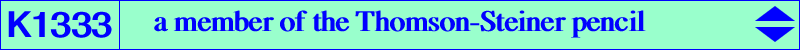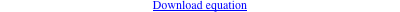too complicated to be written here. Click on the link to download a text file.X(2), X(3), X(99), X(187), X(574), X(11676), X(39162), X(39163), X(39164), X(39165), X(55164), X(55165) foci of the Steiner inellipse vertices of the Thomson triangle antipodes of points of K422 = pK(X6, X5999) on (O), these points lie on K017 infinite points of K751 = pK(X6, X9855) vertices of the 1st and reflected 1st Brocard triangles Geometric properties :See Table 81 for general properties. K1333 is the cubic F(T) obtained when T = 4 / (3 - cot2ω) where ω is the Brocard angle.